# Programming with Matlab: files, loops, conditionals, etc.

In this practice we will focus essentially on the aspects of Matlab linked to programming. Matlab uses its own high-level language that is compiled with C ++ (which is properly the development language). The fact that it is a compiled language means that if we do not do things right the speed of calculation can be significantly reduced.

## Matlab File Types: scripts and functions

Matlab files have the extension .m and can be classified into two types, function files and scripts.
• Script files: Script files are simply files with instruction lines with Matlab that run in the order they are written.
• Function files: These are files that are designed to be called from script files to make a certain calculation from input variables and return output variables. Function files are very easily recognized because they must start with the keyword function.
The syntax of a function file is:
[out1, out2,...] = functionName (input1,input2,...)
Where the input values are the input variables and the output variables.
Note: Function files must be named as the function name. So, for example, maxFunc.m will be the name of the file that contains the maxFunc function.
Example: Suppose that, as an example, we want to calculate the maximum between two functions when we evaluate them at an x-point. We will do this for these two functions: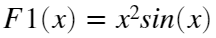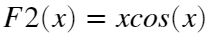This forces us to create a script that initializes the value of x and calls the function. We will need to create the function, give it a name, and define the input and output variables. The script file would be: (must be created, named and saved)
x=0.2;
[maxF,indFun] = maximFunc(x); %call the function maximFunc
fprintf('For x= %e, the max= %e value, for the function %d \n',x,maxF,indFun)
For x= 2.000000e-01, the max= 1.960133e-01 value, for the function 2
%
% The file for the function must be named maximFunc.m and it can be found at the end of this page
%

## Loops: for and while

The two options for looping in a Matlab program are (as in most programming languages) the for and while loops.
As a general rule we will use a for loop if we know exactly how many times we will do the loop (such as traversing an array or vector, etc.). If the number of iterations depends on a condition and therefore it is not clear how many iterations will be needed, then we will use a while loop.
The for always usually has a counter that updates automatically, while the while needs to do so explicitly.
%------ for example:
x=1:10;
x=x/norm(x); %normalize the array
suma=0;
for i=1:10
suma=suma+x(i)^2;
end
norma=sqrt(suma) % make the sum in a 'manual' way
norma = 1
%------ while example:
n=1;
S(1)=1;
while (abs(S(n)-pi^2/6) > 1.e-4)
n=n+1;
S(n)=S(n-1)+1/n^2;
end
n
n = 10000

## Exercise 1:

Add all the components of an mxn array: A = rand (m, n) using two for loops one for rows and the other for columns. Compare the result with the Matlab sum (A (:)) statement.

## Exercise 2:

Use a while to calculate the number of iterations required for the terms of the sequence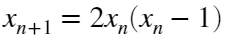, taking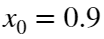until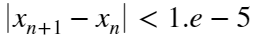## Conditionals: if, else, elseif

When programming an algorithm, conditionals are essential. For the simplest case (two options), in Matlab the syntax is
• if (condition)
do some computations
• else (condition)
do some other computations
• end
x=-3; %compute the absolute value
if (x > 0)
x = x;
else
x=-x;
end
If there are consecutive (nested) conditions then the syntax is:
• if (condition)
do some computations
• elseif (condition)
do some other computations
...............
• elseif (condition)
do some other computations
• end
x=rand(); %random value in [0,1]
if (x < 0.3)
val = 1;
elseif((x >= 0.3) && (x < 0.6)) %two simultaneous conditions
val = 2;
elseif((x >= 0.6) && (x <= 1))
val = 3;
end
[x, val]
ans = 1×2
0.8147 3.0000

## Needed Functions:

function [maxF, indFun]=maximFunc(x)
y1 = x^2*sin(x); %only scalar values can be computed
y2 = x*cos(x);
[maxim, ind] = max([y1,y2]); %the max function give us all the information
maxF = maxim;
indFun = ind;
end